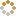﻿ N-B-U-T Online Judge ::  矩阵链乘法
• ###  矩阵链乘法

• 时间限制: 1000 ms　内存限制: 65535 K
• 问题描述
• 给定一个有n个矩阵的矩阵链A1A2A3…An，其中矩阵Ai(i=1,2,3…n)的维度为pi-1*pi。我们知道，两个维度分别为m*r和r*n的矩阵用一般的矩阵乘法相乘，所需的运算次数为m*r*n，最后得到一个维度为m*n的结果矩阵。对于矩阵链问题，因为矩阵乘法具有结合律，其运算顺序有很多中选择。换句话说，不论如何括号其乘积，最后结果都会是一样的。例如，若有四个矩阵A、B、C和D，将可以有：

```(ABC)D = (AB)(CD) = A(BCD) = A(BC)D = ...
```

但括号其乘积的顺序会影响到需要计算乘积所需简单算术运算的数目，即其效率。例如，设A为一10*30矩阵，B为30*5矩阵与C为5*60矩阵，则:

```(AB)C有(10*30*5) + (10*5*60) = 1500 + 3000 = 4500 个运算
A(BC)有(30*5*60) + (10*30*60) = 9000 + 18000 = 27000 个运算
...
```

明显地，第一种方式要有效多了。所以，矩阵链乘法问题也就是如何对矩阵乘积加括号，使得它们的乘法次数达到最少。

• 输入
• 输入的第一行为一个正整数n(1<=n<=200)。表示矩阵的个数。
输入的第二行包含n+1个整数，分别表示pi(0<=i<=n)，其中每个pi在[1,200]范围内。
• 输出
• 输出一个整数表示最少要进行的乘法次数。
• 样例输入
• ```3
1 2 3 4
3
10 30 5 60
```
• 样例输出
• ```18
4500
```
• 提示
• `无`
• 来源
• `Timebug`
• 操作
﻿